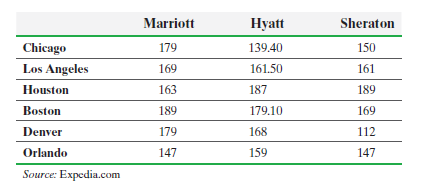×
Get Full Access to Statistics: Informed Decisions Using Data - 5 Edition - Chapter 13.3 - Problem 10
Get Full Access to Statistics: Informed Decisions Using Data - 5 Edition - Chapter 13.3 - Problem 10

×

# ?Lodging A travel agent wanted to know whether the price (in dollars) of Marriott, Hyatt, and Sheraton Hotels differed significantly. She knew that locISBN: 9780134133539 240

## Solution for problem 10 Chapter 13.3

Statistics: Informed Decisions Using Data | 5th Edition

• Textbook Solutions
• 2901 Step-by-step solutions solved by professors and subject experts
• Get 24/7 help from StudySoup virtual teaching assistantsStatistics: Informed Decisions Using Data | 5th Edition

4 5 1 395 Reviews
24
1
Problem 10

Lodging A travel agent wanted to know whether the price (in dollars) of Marriott, Hyatt, and Sheraton Hotels differed significantly. She knew that location of the hotel is a factor in determining price, so she blocked each hotel by location. After randomly selecting six cities and obtaining the room rate for each hotel, she obtained the following data:(a) Normal probability plots for each treatment indicate that the requirement of normality is satisfied. Verify that the requirement of equal population variances for each treatment is satisfied.

(b) Is there sufficient evidence that the mean cost of the room is different among the three hotel chains at the a = 0.05 level of significance?

(c) If the null hypothesis from part (b) was rejected, use Tukey’s test to determine which pairwise means differ using a familywise error rate of a = 0.05.

Step-by-Step Solution:

Step 1 of 5) Lodging A travel agent wanted to know whether the price (in dollars) of Marriott, Hyatt, and Sheraton Hotels differed significantly. She knew that location of the hotel is a factor in determining price, so she blocked each hotel by location. After randomly selecting six cities and obtaining the room rate for each hotel, she obtained the following data: (a) Normal probability plots for each treatment indicate that the requirement of normality is satisfied. Verify that the requirement of equal population variances for each treatment is satisfied. (b) Is there sufficient evidence that the mean cost of the room is different among the three hotel chains at the a = 0.05 level of significance (c) If the null hypothesis from part (b) was rejected, use Tukey’s test to determine which pairwise means differ using a familywise error rate of a = 0.05. To test any one of these hypotheses, we need to know the sampling distribution of b1.

Step 2 of 2

##### ISBN: 9780134133539

The full step-by-step solution to problem: 10 from chapter: 13.3 was answered by , our top Statistics solution expert on 01/15/18, 03:19PM. Statistics: Informed Decisions Using Data was written by and is associated to the ISBN: 9780134133539. The answer to “?Lodging A travel agent wanted to know whether the price (in dollars) of Marriott, Hyatt, and Sheraton Hotels differed significantly. She knew that location of the hotel is a factor in determining price, so she blocked each hotel by location. After randomly selecting six cities and obtaining the room rate for each hotel, she obtained the following data: (a) Normal probability plots for each treatment indicate that the requirement of normality is satisfied. Verify that the requirement of equal population variances for each treatment is satisfied.(b) Is there sufficient evidence that the mean cost of the room is different among the three hotel chains at the a = 0.05 level of significance?(c) If the null hypothesis from part (b) was rejected, use Tukey’s test to determine which pairwise means differ using a familywise error rate of a = 0.05.” is broken down into a number of easy to follow steps, and 139 words. This full solution covers the following key subjects: . This expansive textbook survival guide covers 88 chapters, and 2422 solutions. Since the solution to 10 from 13.3 chapter was answered, more than 210 students have viewed the full step-by-step answer. This textbook survival guide was created for the textbook: Statistics: Informed Decisions Using Data, edition: 5.

## Discover and learn what students are asking

Calculus: Early Transcendental Functions : Conics, Parametric Equations, and Polar Coordinates
?In Exercises 1–6, match the equation with the correct graph. [The graphs are labeled (a), (b), (c), (d), (e), and (f).]

Calculus: Early Transcendental Functions : Graphs and Models
?In Exercises 5-14, sketch the graph of the equation by point plotting. $$y=4-x^{2}$$

Statistics: Informed Decisions Using Data : The Design of Experiments
?Explain the difference between a single-blind and a double- blind experiment.

Statistics: Informed Decisions Using Data : Testing the Significance of the Least-Squares Regression Model
?The U.S. Population The following data represent the population of the United States for the years 1900–2010 An ecologist i

Unlock Textbook Solution